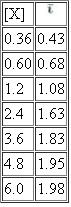# Modern Experimental Biochemistry

Chemistry

## Quiz 3 : Using Gel Filtration to Study Ligand-Protein InteractionsStudy FlashcardsLooking for Biochemistry Homework Help?

## Quiz 3 :Using Gel Filtration to Study Ligand-Protein Interactions

Question TypeWithout looking back in the experiment, write a list of four natural or synthetic molecules that may be transported by human serum albumin.
Free
Essay

In this problem, we are asked to supply a list of four natural of synthetic molecules that can be transported by albumin.
As a transport protein, it is the job of albumin to bind a variety of molecules and move them from their point of origin (digestive tract, lungs, intravenous introduction, etc) to the location where they will have their action. Albumin does this by creating non-covalent interactions with the material, binding it up, and then moving it through the blood until it reaches the location where it should take effect - removal of the material from albumin is often a matter of diffusion driving dissociation from the albumin into the serum at a new location.
Natural materials that can be transported by albumin include fatty acids, bilirubin, thyroxine, and a number of steroid hormones. Synthetic molecules include aspirin (acetylsalicylic acid), sulfaniliamide compounds, clofibrate, and digitalis - a molecule with cardiac functions.

Tags
Choose question tagEquation E3.5 in this experiment can be used to determine Krvalues, but hyperbolic plots are obtained. Can you convert Equation E3.5 into an equation that will yield a linear plot without going through all the changes necessary for the Scatchard equation? Hint: Study the conversion of the Michaelis-Menten equation to the Lineweaver-Burk equation.
Free
Essay

In this problem, we are asked to derive a linear relationship from the following equation: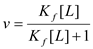This equation would be used to discussion speed of an enzyme reaction in relation to the point at which an enzyme L reaches half of its saturation point - this term is denoted as K f. If we graph this equation as is, we will receive an unusual curve, which will be hard to relate to other processes and difficult to assess intersections. By taking the reciprocal of this equation and using some algebraic rearrangement, we can turn this equation into a linear representation.
We begin with our equation: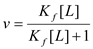We take the reciprocal and end up with: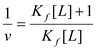We rearrange, and end up with: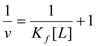Tags
Choose question tagBelow is a table showing the % binding of phenol red to human serum albumin versus pH of the reaction mixture. Draw a graph showing the results and explain the shape of the curve.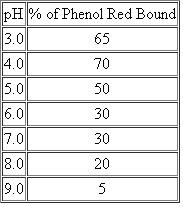Free
Essay

In this problem, we are asked to graph the following data on phenol red binding and provide an explanation for the trend.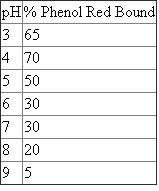The data above becomes the following graph: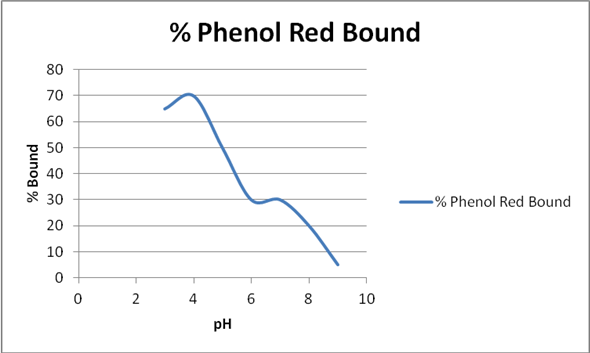As the pH increases, the affinity of phenol red for binding decreases; therefore, phenol red's binding is pH-dependent.

Tags
Choose question tag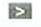Amino acids and fatty acids do not readily elute from gas chromatography columns even at temperatures above 200°C. What can be done to these biomolecules to allow gas chromatographic analysis?

There is no answer for this question

Tags
Choose question tagA research project you are working on involves the study of sugar binding to human albumin. The sugars to be tested are not fluorescent, and you do not wish to use a secondary fluorescent probe. Human albumin has only one tryptophan residue, and you know that this amino acid is fluorescent. You find that the tryptophan fluorescence spectrum of human albumin undergoes changes when various sugars are added. Can you explain the results of this experiment and discuss the significance of the finding?
Essay
Tags
Choose question tagDescribe the various detection methods that can be used in HPLC. What types of biomolecules are detected by each method?
Essay
Tags
Choose question tagThe binding of a ligand, L, to a protein is studied as described in this experiment. The following data are collected using 7.5 × 10 - 6 molar protein.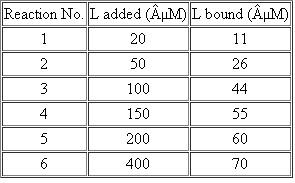Use a graphical analysis to determine n and K f.
Essay
Tags
Choose question tagThe Bradford protein assay as described in Chapter 2 is based on the absorbance change that occurs upon binding of Coomassie Blue dye to proteins. Explain how you would study the dynamics of this binding process and experimentally determine the number of binding sites on a protein.
Essay
Tags
Choose question tagExplain how you could use a gel filtration column to remove sodium chloride from a solution of bovine serum albumin. Draw a graph representing the elution curve for separation. Assume that two measurements were made on each fraction, a test for the presence of chloride and absorbance at 280 nm.

There is no answer for this question

Tags
Choose question tagDescribe how you would use "affinity elution" to remove the enzyme alcohol dehydrogenase bound to a Cibracron blue-agarose column.
Essay
Tags
Choose question tag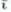Draw the elution curve ( A 280 vs. fraction number) obtained by passing a mixture of the following proteins through a column of Sephadex G-100. The molecular weight is given for each protein.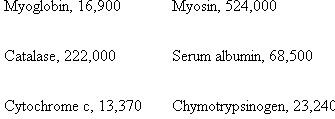Essay
Tags
Choose question tagExplain the elution order of amino acids in Figure 3.13.
Essay
Tags
Choose question tagName three enzymes that you predict will bind to the affinity support, 5?-AMP-agarose.

There is no answer for this question

Tags
Choose question tagIn your biochemistry research project you find that the binding of a ligand to a protein decreases if the ionic strength of the buffer solvent is increased. What tvpe of noncovalent bonding might be involved in the ligand-protein complex?
Essay
Tags
Choose question tagExplain how your experimental results would change if you did the phenol red-BSA binding studies with added stearic acid.
Essay
Tags
Choose question tagBriefly describe how you would experimentally measure the exclusion limit for a Sephadex gel whose bottle has lost its label.
Essay
Tags
Choose question tagAmino acid analyzers are instruments that automatically separate amino acids by cation-exchange chromatography. Predict the order of elution (first to last) for each of the following sets of amino acids at pH = 4. (a) Gly, Asp, His (b) Arg, Glu, Ala (c) Phe, His, Glu
Essay
Tags
Choose question tagIn what order would the following proteins be eluted from a DEAE-cellulose ion exchanger by an increasing salt gradient. The pH 1 is listed for each protein.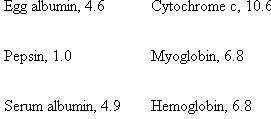Essay
Tags
Choose question tagPredict the relative order of paper chromatography R f values for the amino acids in the following mixture: Ser, Lys, Leu, Val, and Ala. Assume that the developing solvent is n -butanol, water, and acetic acid.
EssayA drug, X, has a strong affinity for serum albumin. When X was bound to albumin, an increase in absorbance was noted. Thevalues were determined from these absorbance measurements. Use the data below to determine K f and n for the interaction between X and albumin. Prepare two types of graphs and compare the results. In one graph plotversus [X], and in the second plot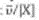versus. Which is the better method for calculating binding constants? Why?